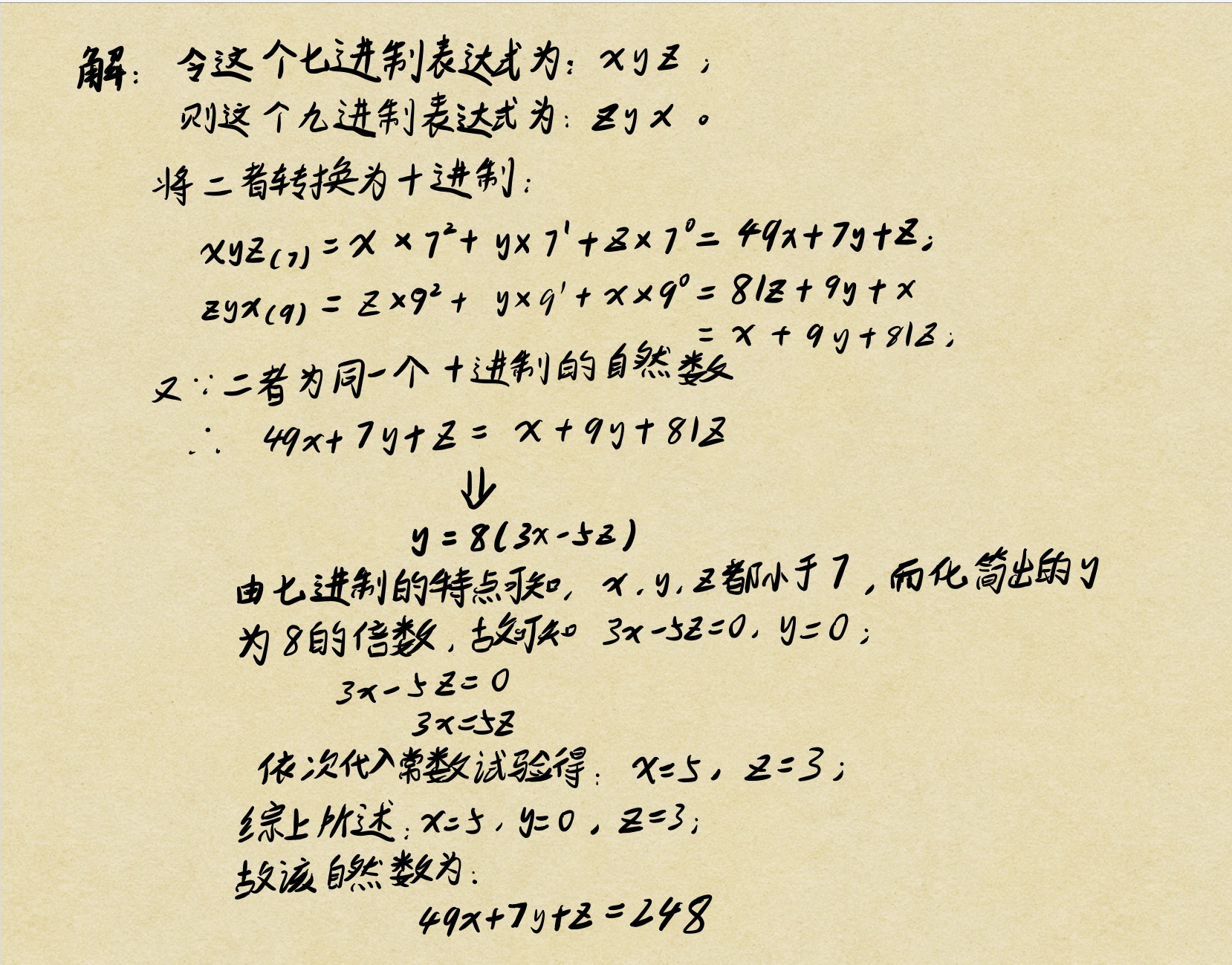# 一个自然数的七进制表达式是一个三位数，而这个自然数的九进制表示也是一个三位数，且这两个三位数的数码顺序正好相反，求这个三位数。for(i=1;i<7;i++)
for(j=0;j<7;j++)
for(k=1;k<7;k++)
if(i*9*9+j*9+k==i+j*7+k*7*7)


#include<stdio.h>
int main()
{
int i,j,k;
for(i=1;i<7;i++)
for(j=0;j<7;j++)
for(k=1;k<7;k++)
if(i+j*7+k*7*7==i*9*9+j*9+k)
{
printf("这个奇异的三位数是(括号内是进制):\n");
printf("%d%d%d(7)=%d%d%d(9)=%d(10)\n",k,j,i,i,j,k,i*9*9+j*9+k);
}
}


05-04335908-101238
11-162892
02-27623
02-24116
01-291272
01-241096
07-29603
07-29624
11-13833